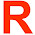### Data models

In database designing we have to consider the data model as an important aspect, in this post we are going to see about the data models and its categories.

### Data models

The structure of a database is the data model. The data model is a,
1. Collection of conceptual tools for describing data,
2. Data relationships,
3. Data semantics,
4. Consistency constraints.

Data model provides a way to describe the design of a database at the physical level, logical level and View level (DBMS views).

### Categories of data model:

The data models can be classified into four different categories they are,
1. Relational Model.
2.  The Entity-Relationship Model (E-R Model)
3.  Object-Based data Model.
4.  Semi-structured Data Model.

### Relational model:

The relational model uses a collection of tables to represent both data and the relationships among those data. We know that a table is a collection of rows and columns and each column has a unique name. The relational model is a type of record-based model.

### Record-based model:

In record-based model,
1. The database is structured in fixed-format records of several types.
2. Each table contains a records (data) of a particular type.
3. Each record type (data type) defines a fixed number of fields (columns) or attributes.
There are three types in this record based data model, they are,
1. Relational Data Model.
2.  Hierarchical Data Model.
3.  Network Data Model.
The Hierarchical and Network Data Model details are available here..

### Database implementation:

The relational model is mostly implemented by using the SQL (Structure Query Languages), let us see about some of the advantages and disadvantages of using SQL.

The advantages of relational data model are given below.
1. Easy to store and retrieve data.
2. Easy execute complex queries.
3. Flexibility.
4. Security.
5. Avoid Data duplication.

1.  Performance.
2.  Storage.

### The entity-relationship model (E-R model):

#### Entity:

An entity is a 'thing' or 'object' in the real world that is distinguishable from other entities. Its better to use entity instead of object since in our future posts we are going use the same. The entity-relationship (E-R) data model is based on a perception of a real world that consist of a collection of basic entities or objects and of relationships among these objects.

### Database Implementation:

The Entity-Relationship model is implemented by using the ERROL query language. ERROL’s semantics and implementation are based on Reshaped Relational Algebra (RRA).

1.  Simplicity.
2.  Design Visualization.
3.  Integration with Relational Model.

1. Loss of information.
2.  No representation of data manipulation.

### Object-Oriented Data Model:

The Object-Oriented data model is the extension of E-R (Entity-Relationship) data model with notions of Object-Oriented concepts such as,
1. Encapsulation.
2. Functions (Methods).
3. Object identity.
The Object-Relational data model combines features of the Object-Oriented data model and Relational data model.

#### Database Implementation:

The Object-Oriented data model can be implemented by using the object oriented programming languages such as  Delphi, Ruby, Python, Perl, Java, C#, Visual Basic .NET, C++, Objective-C and Small talk.

1. Good performance.
2. Good communication between users, analysts, designers and programmers.
3. Consistency.
4. Robustness.
5. Re-usability.

1. Difficult to use.
2. Does not allow existing systems.
3. No Data abstraction.

### Semi-structured Data Model:

The Semi-structured data model is totally differ from the remaining data models, in which specification of data is implemented, where individual data items of the same type may have different sets of attributes.

#### Database Implementation:

Extensible Markup Language (XML) is used to represent Semi-Structured data.

1. Flexible.
2. Communication between databases.

1. No clear separation between the data and schema.
2. Possibility of losing data constraints.
I hope this post provides an basic understanding of the data modeling, in the future post, let us see data modeling in more details.

1.Interesting....

1.Thank you buddy.

2.Ya this is very useful for me rathish......

3.Thank you for your interest on this post.